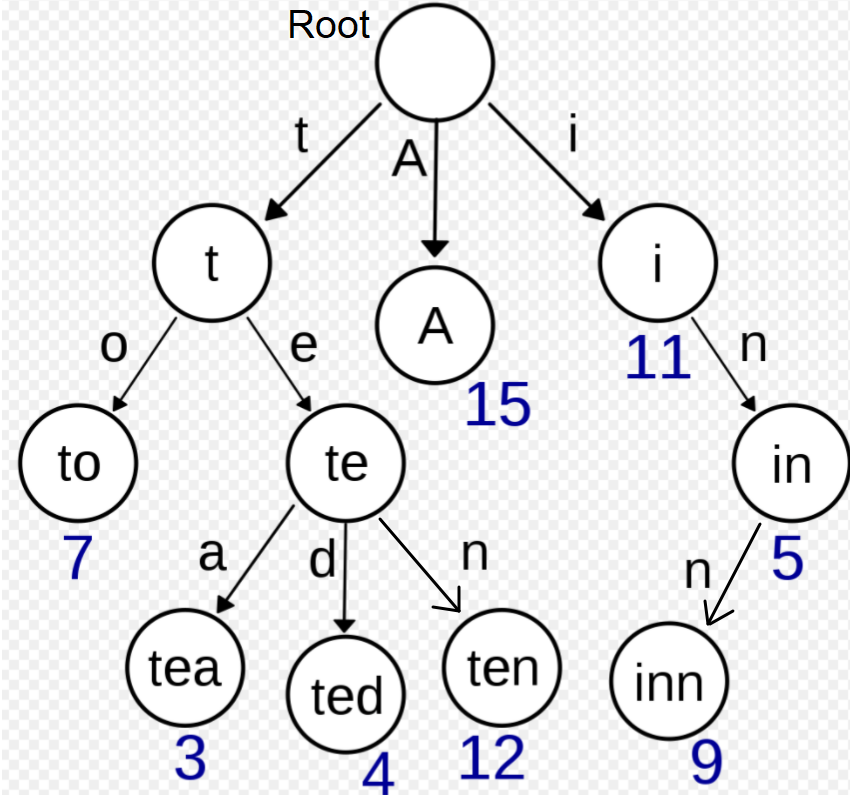# Introduction and implementation of Trie

## Introduction:

Trie is a ‘Tree ’-based data structure mainly used to search words efficiently from an array of Strings or Dictionary. Trie consists of nodes, where each node represents one alphabet, and Trie stores the entire word using these nodes.

Trie is also known as the ‘Prefix Tree.’

Properties of Trie Data Structure:

1. The root node of the trie is always null.
2. All the children of nodes are sorted alphabetically.
3. Every node can have a maximum of 26 children (A to Z).
4. Every node (except the root) can store one letter of the alphabet

## Representation of a Trie Node:

A ‘TrieNode’ class consists of the following things:

1. An array of TrieNodes of size 26. Each index represents one character from 26 English alphabets(A - Z).
2. A boolean variable ‘isEnd’ representing if this is the last level of your current word.Source: wikipedia

In the above picture -  to, tea, ted, ten, inn, A are stored in the Trie.

## Operations in a Trie:

There are three operations we can perform in a Trie:

1. Insertion of a word
2. Searching of a word
3. Deletion of a word

## Insertion of a word in a Trie:

1. Every word starts inserting below the root level.
2. If the present character node is already present in the Trie, use that node to form your word. But, if there is no present character node at the current level, make a new TrieNode and add its reference to its parent’s TrieNode array. Remember, in the latter case, you need to update the ‘isEnd’ boolean also.
3. The word’s length determines the depth of the Trie Tree.

## Searching a word in a Trie:

We start comparing the characters of the words with the nodes present in the Trie. Now, there can be the following two cases:

1. If there is no node present for the current character at any moment, that means the current word is not present in the Trie.
2. Suppose you reach the end of your word. You need to check if ‘isEnd’ for your current node is set to ‘True’ or ‘False.’ If it’s true, that means the word is present in the Trie else no.

## Deleting a Word in a Trie:

In trie, we delete any word in a bottom-up manner consider the following cases:

1. If the current word is not present in the Trie, your delete operation should not modify the Trie.
2. If the current word is present as the prefix to another word, just update the ‘isEnd’ boolean variable of the last character node of your current word.
3. If the current word is not present as the prefix to any word, delete all the references of the character’s node in the Trie from the bottom until you find a Node with its ‘isEnd’ variable set to true.

### Implementation:

#### Time Complexity:

All the operations, including Insert, Delete, and Search function works in O(Word_Length) time because we iterate the entire word and keep inserting the node for each node if it is not present. And as soon we iterate the entire word, out word gets inserted, deleted or searched.

#### Space Complexity:

Implementing trie takes O(Alphabet_Size * numberOfWords * Word_Length) because we are inserting each word in the Trie and each alphabet of the word takes up some memory.

## FAQs:

1. How much time does it take to insert all the words in the Trie Data Structure?
Time taken to insert all the words in the Trie is O(N * M) where ‘N’ is a total number of words and ‘M’ is the largest length of any word because insertion of a single word takes O(M) time where M is the length of a word. Hence inserting ‘N’ words will take O(N * M) time.

2. What is the main advantage of Tries?
We can insert and search any string in the trie in O(word_length) time.

3. What is the main disadvantage of Trie?
Trie takes a lot of memory to store all the Strings.

## Key TakeAways:

In this blog, we have covered the following things:

1. What operations we can perform on a Trie.
2. How to implement all three functions of Trie.
3. Time Complexity and Space Complexity of Trie.

Until then, All the best for your future endeavors, and Keep Coding.BY: NISHANT RANA